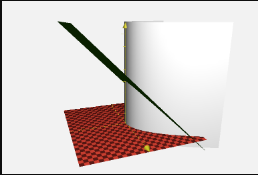# The solid enclosed by the cylinder y=x^2 and the planes z=0 and y+z=1

The solid enclosed by the cylinder y=x^2 and the planes z=0 and y+z=1